# Texas Go Math Grade 3 Lesson 10.5 Answer Key Model with Arrays

Refer to our Texas Go Math Grade 3 Answer Key Pdf to score good marks in the exams. Test yourself by practicing the problems from Texas Go Math Grade 3 Lesson 10.5 Answer Key Model with Arrays.

## Texas Go Math Grade 3 Lesson 10.5 Answer Key Model with Arrays

Essential Question
How can you use arrays to solve division problems?
An arrangement of objects, pictures, or numbers in columns and rows is called an array, and division of the objects or pictures in equal group solve the problem.

Investigate
Materials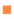square tiles
You can use arrays to model division and find equal groups.

A. Count out 30 tiles. Make an array to find how many rows of 5 are in 30.6 rows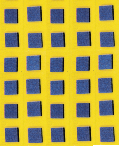Explanation:
30 tiles are grouped 5 of 6 rows
30÷5 = 6

B. Make a row of 5 tiles.Explanation:
The array contains 30 tiles which are grouped 5 of 6 rows.
30÷5 = 6

C. Continue to make as many rows of 5 tiles as you can.
How many rows of 5 did you make? ____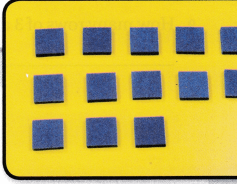6 rows
Explanation:
30÷5 = 6
So, we can make 6 rows.

Make Connections
You can write a division equation to show how many rows of 5 are in 30. Show the array you made in Investigate by completing the drawing below.30 ÷ 5 = ___
There are ___ rows of 5 tiles in 30.
So, 30 ÷ 5 = _____
completing the drawing:30 ÷ 5 = 6
There are 6 rows of 5 tiles in 30.
So, 30 ÷ 5 = 6

Math Idea
You can divide to find the number of equal rows or to find the number in each row.
n x n
1 x 1
2 x 2
3 x 3

Share and Show

Use square tiles to make an array. Solve.

Question 1.
How many rows of 3 are in 18?
6 rows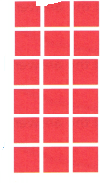Explanation:
Array has 18 tiles arranged in rows.
Each row has 3 tiles.
Total rows in array
18 ÷ 3 = 6

Question 2.
How many rows of 6 are in 12?
2 rowsExplanation:
Array has 12 tiles arranged in rows.
Each row has 6 tiles.
Total rows in array
18 ÷ 6 = 2

Question 3.
How many rows of 7 are in 21?
3 rowsExplanation:
Array has 21 tiles arranged in rows.
Each row has 7 tiles.
Total rows in array
21 ÷ 7 = 3

Question 4.
How many rows of 8 are in 32?
4 rows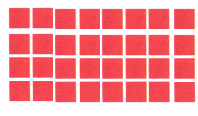Explanation:
Array has 32 tiles arranged in rows.
Each row has 8 tiles.
Total rows in array
32 ÷ 8 = 4

Make an array. Then write a division equation.

Question 5.
25 tiles in 5 rows
5 tiles in a row
25÷5 = 5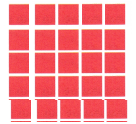Explanation:
Array has 25 tiles arranged in rows.
Total 5 rows in array
Each row has 25 ÷ 5 = 5

Question 6.
14 tiles in 2 rows
7 tiles in a row
14÷2 = 7Explanation:
Array has 14 tiles arranged in rows.
Each row has 7 tiles
Total rows in array 14 ÷ 2 = 7

Question 7.
28 tiles in 4 rows
7 tiles in a row
28÷4 = 7Explanation:
Array has 28 tiles arranged in rows.
Total 4 rows in array
Each row has 28 ÷ 7 = 7

Question 8.
27 tiles in 9 rows
3 tiles in a row
27÷9=3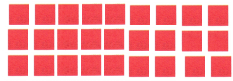Explanation:
Array has 27 tiles arranged in rows.
Total 9 rows in array
Each row has 27 ÷ 9 = 3

Question 9.
How many rows of 3 are in 15?
5 rows
15 ÷ 5 = 3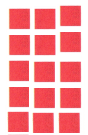Explanation:
Array has 15 tiles arranged in rows.
Each row has 3 tiles
Total rows in array 27 ÷ 9 = 3

Question 10.
How many rows of 8 are in 24?
3 rows
24 ÷ 8 = 3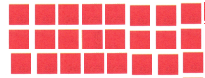Explanation:
Array has 24 tiles arranged in rows.
Each row has 8 tiles
Total rows in array 24 ÷ 8 = 3

Math Talk
Mathematical Processes
Explain when you Count the number of rows to find the answer and when you count the number of tiles in each row to find the answer.
To find the number of tiles in a row,  total tiles ÷ number of row is calculated.
To find the number of rows,  total tiles ÷ number of tiles in row is calculated.

Question 11.
Write Math Show two ways you could make an array with tiles for 18 ÷ 6. Shade squares on the grid to record the arrays.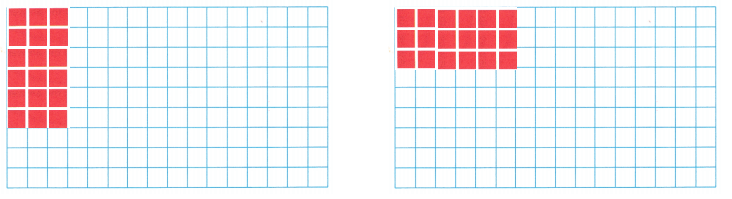18 ÷ 6 = 3
6 rows of 3 tiles
or
3 rows of 6 tiles
Explanation:
count the number of tiles on the grid and shade as,
6 rows of 3 tiles
3 rows of 6 tiles

Problem Solving

Question 12.
Thomas has 28 tomato seedlings to plant in his garden. He wants to plant 7 seedlings in each row. How many rows of tomato seedlings will Thomas plant?
4 rows of tomato seedlings.
Explanation:
Thomas has 28 tomato seedlings to plant in his garden.
He wants to plant 7 seedlings in each row.
Total rows of tomato seedlings Thomas plant
28 ÷ 7 = 4

Question 13.
H.O.T. Use Math Language Tell how to use an array to find how many rows of 8 are in 40.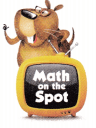An array is an orderly arrangement (often in rows, columns or a matrix) that is most commonly used as a visual tool for demonstrating multiplication and division .
40 ÷ 8 = 5
Explanation:
An array refers to a set of numbers or objects that will follow a specific pattern.40 ÷ 8 = 5

Question 14.
Faith plants 36 flowers in 6 equal rows. How many flowers are in each row?
6 flowers in each row.
Explanation:
Faith plants 36 flowers in 6 equal rows.
Total flowers in each row
36 ÷ 6 = 6

Question 15.
H.O.T. Multi-Step There were 20 plants sold at a store on Saturday and 30 plants sold at the store on Sunday. Customers bought 5 plants each. How many customers in all bought the plants?
10 customers bought the plants.
Explanation:
There were 20 plants sold at a store on Saturday and
30 plants sold at the store on Sunday.
Total plants sold on both days 20 + 30 = 50
Customers bought 5 plants each.
Number of customers bought the plants
50 ÷ 5 =10

Question 16.
H.O.T. Multi-Step Lionel made an array with 24 tiles. The number of rows is 5 more than the number of tiles in each row. How many rows are in Lionel’s array?
3 rows
Explanation:Lionel made an array with 24 tiles.
The number of rows is 5 more than the number of tiles in each row.
Number of rows in Lionel’s array is 3

Fill in the bubble for the correct answer choice.
You may use objects or models to solve.

Question 17.
Multi-Step Mrs. Weston is baking 24 oatmeal raisin cookies. There will be 4 rows of cookies on the cookie tray. She has already put one row of cookies on the tray. How many more cookies does she have left to put on the tray?
(A) 6
(B) 20
(C) 18
(D) 3
Mrs. Weston is baking 24 oatmeal raisin cookies.
24 ÷ 4 = 6
4 – 1 = 3 rows left
3 x 6 = 18
18 more cookies she have left to put on the tray.

Question 18.
Representations Which division equation is shown by the array?(A) 24 ÷ 3 = 8
(B) 24 ÷ 4 = 6
(C) 24 ÷ 2 = 12
(D) 21 ÷ 3 = 7
Option (A)
Explanation:
The array contains 24 tiles,
arranged as 3 rows of 8 tiles.

Question 19.
Multi-Step Hajune finds 9 black rocks one week and 9 black rocks the next week. He wants to keep the rocks in a box with 3 rows. How many black rocks can he put in each row?
(A) 18
(B) 6
(C) 12
(D) 3
6 black rocks in a box.
Explanation:
Hajune finds 9 black rocks one week and 9 black rocks the next week.
Total black rocks 9 + 9 = 18
He wants to keep the rocks in a box with 3 rows.
Total black rocks he put in each row
18 ÷ 3 = 6

Texas Test Prep

Question 20.
Sally makes an array using 36 coins. If she puts 4 coins in each row, how many rows does she make?
(A) 40
(B) 32
(C) 8
(D) 9
Option(D)
Explanation:
Sally makes an array using 36 coins.
If she puts 4 coins in each row,
Total rows she make
36 ÷ 4 = 9

### Texas Go Math Grade 3 Lesson 10.5 Homework and Practice Answer Key

Make an array. Then write a division equation.

Question 1.
21 tiles in 3 rows
7 tiles in each row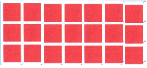21 ÷ 3 = 7
Explanation:
Array has 21 tiles arranged in 3 rows.
Each row has 27 ÷ 9 = 3 tiles

Question 2.
36 tiles in 6 rows
6 tiles in each row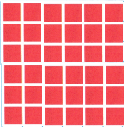36 ÷ 6 = 6
Explanation:
Array has 36 tiles arranged in 6 rows.
Each row has 36 ÷ 6 = 6 tiles
Question 3.
16 tiles in 8 rows
2 tiles in each row16 ÷ 8 = 2
Explanation:
Array has 16 tiles arranged in 8 rows.
Each row has 16 ÷ 8 = 2 tiles

Question 4.
18 tiles in 3 rows
6 tiles in each row
18 ÷ 3 = 6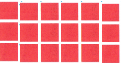Explanation:
Array has 18 tiles arranged in 3 rows.
Each row has 18 ÷ 3 = 6 tiles

Question 5.
How many rows of 4 are in 32?
8 tiles in each row
32 ÷ 4 = 8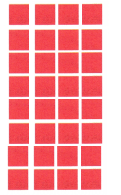Explanation:
Array has 21 tiles arranged.
Each row has 4 tiles
number of rows 27 ÷ 9 = 3

Question 6.
How many rows of 4 are in 16?
4 tiles in each row
16 ÷ 4 = 4Explanation:
Array has 21 tiles arranged.
Each row has 4 tiles
number of rows 27 ÷ 9 = 3

Problem Solving

Question 7.
Hannah has a collection of 27 seashells. She wants to put 9 shells on each shelf. How many shelves does Hannah need?
3 shelves
27 ÷ 9 = 3
Explanation:
Hannah has a collection of 27 seashells.
She wants to put 9 shells on each shelf.
Number of shelves Hannah need
27 ÷ 9 = 3

Question 8.
Dexter buys 18 fish. He wants to put an equal number of fish into 3 fishbowls. How many fish will he put in each fishbowl?
6 fishes in each bowl.
Explanation:
He wants to put an equal number of fish into 3 fishbowls.
Number of fish he put in each fishbowl
18 ÷ 3 = 6

Question 9.
Quentin is making a tile design with 35 tiles. He wants to put the tiles in rows. How can he set up the tiles so there are an equal number of tiles in each row?
35÷7 =5 or
35÷5 =7
Explanation:
Quentin is making a tile design with 35 tiles.
He wants to put the tiles in rows.
He can set up the tiles in 2 ways, an equal number of tiles in each row
35÷7 =5 or 35÷5 =7

Texas Test Prep

Lesson Check

Question 10.
Marla makes this array.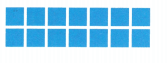What division equation can she write?
(A) 14 ÷ 3 = 7
(B) 14 ÷ 2 = 7
(C) 2 × 7 = 14
(D) 7 + 7 = 14
Option(B)
Explanation:
Marla makes array of 14 tiles, arranged in 2 equal rows.
Number of tiles in each row
14 ÷ 2 = 7

Question 11.
Claude makes this array.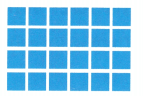What division equation can he write?
(A) 24 ÷ 4 = 6
(B) 24 ÷ 3 = 8
(C) 4 × 6 = 24
(D) 6 × 4 = 20
Option(A)
Explanation:
Claude makes array of 24 tiles.
Each row contains 4 tiles.
Total tiles arranged in equal rows
24 ÷ 4 = 6

Question 12.
Sondra runs for 32 minutes. If she runs 4 miles, how many minutes does it take her to run one mile?
(A) 6 minutes
(B) 7 minutes
(C) 8 minutes
(D) 28 minutes
Option(C)
Explanation:
Sondra runs for 32 minutes.
If she runs 4 miles,
Total minutes it take her to run one mile
32 ÷ 4 = 8

Question 13.
There are 25 students on the playground. The students are in teams of 5. How many teams are on the playground?
(A) 6
(B) 5
(C) 4
(D) 7
Option(B)
Explanation:
There are 25 students on the playground.
The students are in teams of 5.
Total teams on the playground
25 ÷  5 = 5

Question 14.
Multi-Step Jennifer collects 9 animal cards and 21 nature cards. She wants to arrange them on a bulletin board in 6 rows. How many cards will be in each row?
(A) 5
(B) 2
(C) 3
(D) 7
Option(A)
Explanation:
Jennifer collects 9 animal cards and 21 nature cards.
9 + 21 = 30
She wants to arrange them on a bulletin board in 6 rows.
Number of cards in each row
30 ÷ 6 = 5

Question 15.
Multi-Step Zach is planting a garden of 27 tomato plants. He puts the plants into rows of 9. If he has already planted one row, how many more rows does he need to plant?
(A) 3
(B) 2
(C) 18
(D) 4